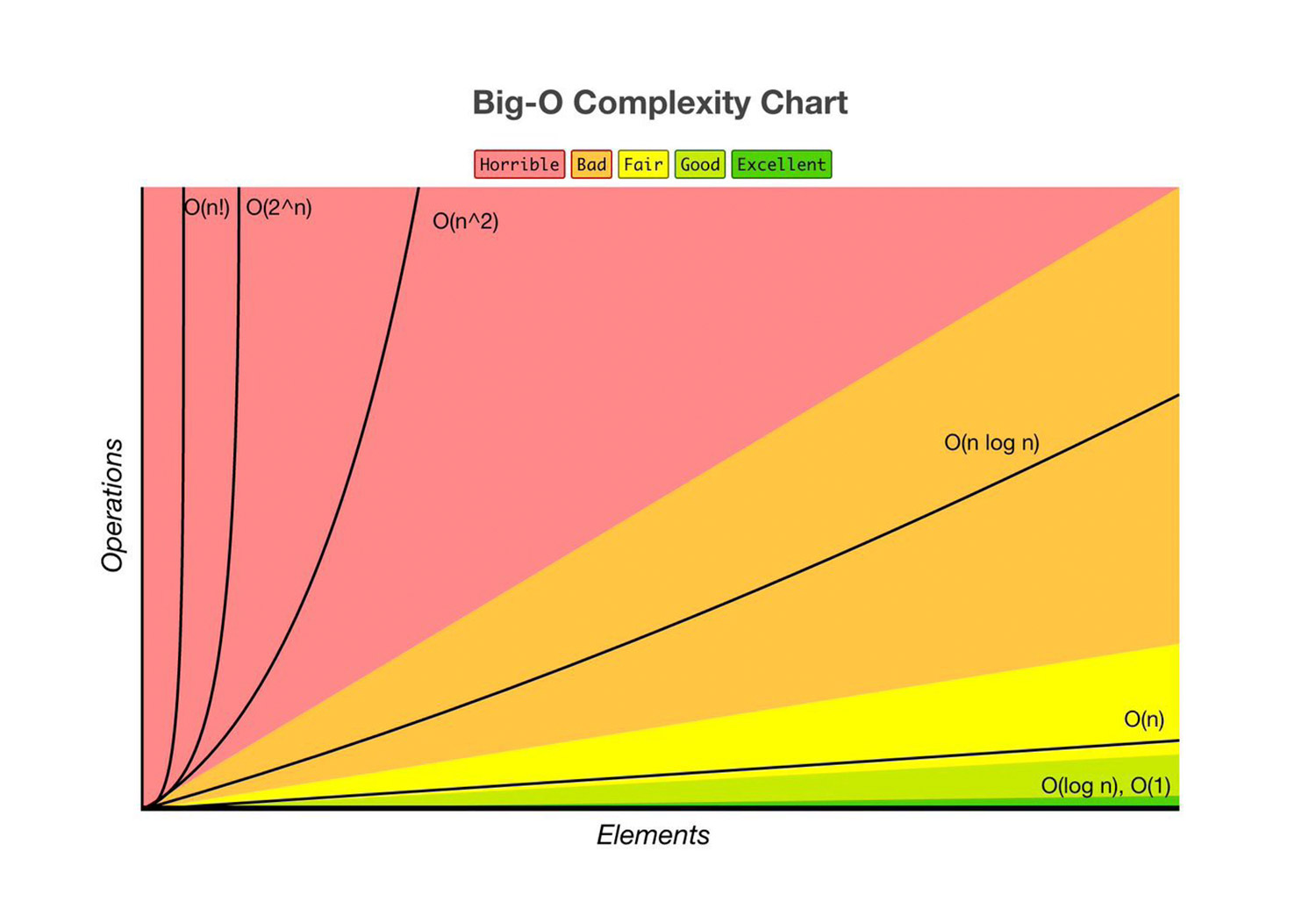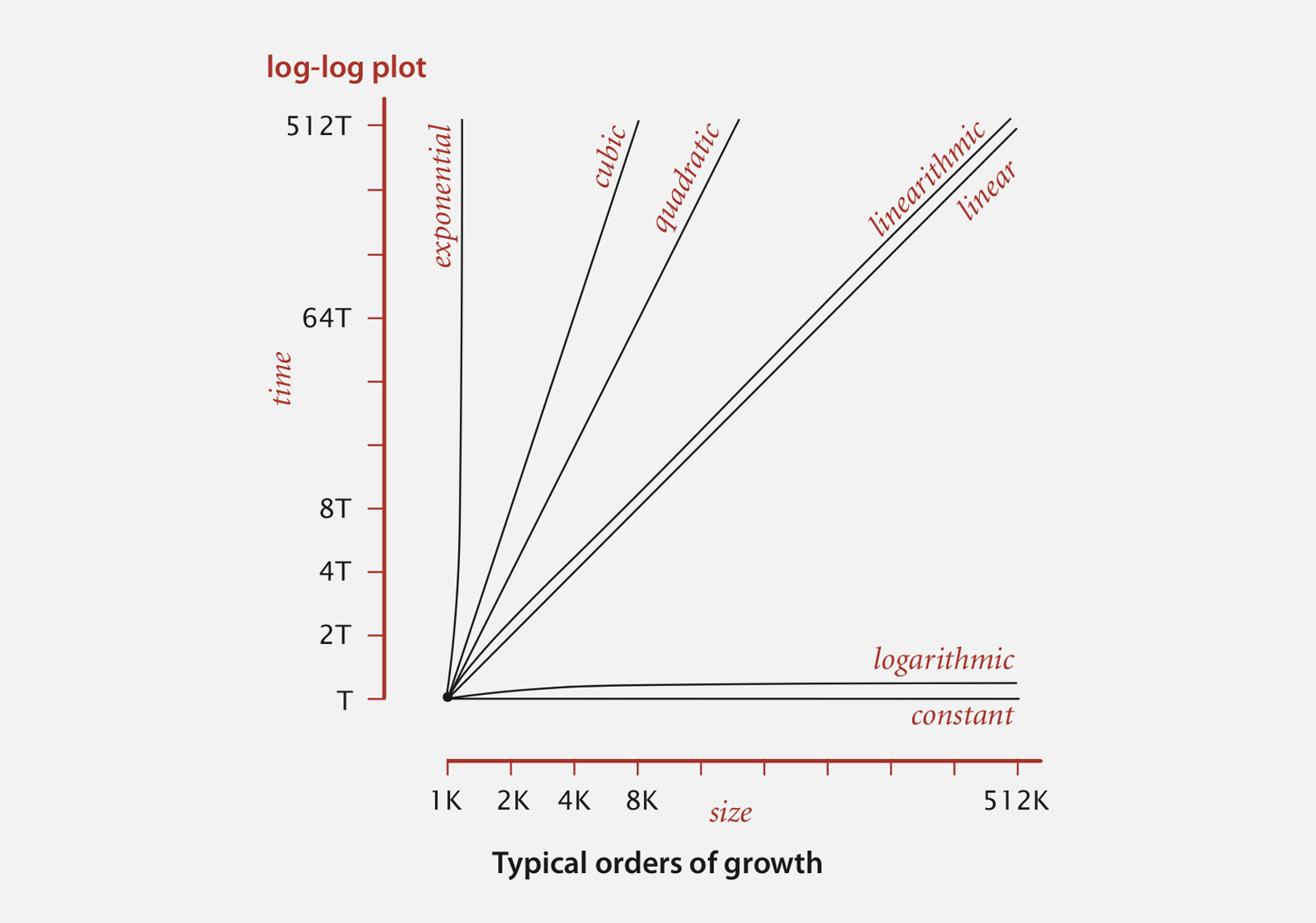publication

# Introduction to Algorithm Complexity Analysis### Miquel Canal

Tuesday 21, April 2020
• Software Engineering
• Big Data

## Algorithm Complexity Analysis

Algorithm Complexity Analysis comes hand in hand with Big O notation. Those are tools that allow programmers to analyse a system and represent its levels of complexity.

The use of theoretical computer science can help developers to improve the overall quality of our code.

When using Algorithm Complexity Analysis, we evaluate how the performance of an algorithm or a logical system changes based on the number of inputs passed to it. If our algorithm takes 1 second to run for an input of size 1000, how will it behave if you double the input size?

Let’s look at an example of an algorithm that stores and array of even numbers based on a given input of numbers:

``````const even = [];
const x = 10;

for ( i = 1; i <= x; i++ ) {
if ( i % 2 == 0 ) {
even.push( i );
}
}``````

To analyse the algorithm, you should find the mathematical representation of the executions as a function. If you take the input as the `n` letter, the above can be described as: `f(n) = 2n`.

The number 2 represents the `if` condition and the `push` execution to store a number. `2n` here means that on each loop we are required to perform those 2 actions.

## Worst Case Scenario

In the example above I have stated that on each loop the algorithm is required to perform 2 actions. However, that is not true as some iterations might not pass the `if` condition.

That is when the worst case scenario comes in. As software engineers we should always look for the worst scenario when analysing. This practice gives us the insight of how an algorithm will perform when executing the most number of instructions to complete.

## Big O notation

This notation is used to reduce the complexity of showing the results of the analysis. Big O notation is easier to read than a mathematical expression. A Big O notation can be expressed like: `O(n)`

And its pronunciation is: “big oh of n”. By using this notation, you can quickly identify that the algorithm complexity of our example depends on the given input (n).

If you compare the mathematical expression `f(n) = 2n` to its Big O notation `O(n)` it is simpler to understand that the algorithm will increase in executions in a direct relation to the input that gets passed by.

The number 2 in our mathematical expression is not taken into account in the Big O notation. The reason behind it that we're interested in the limit of function `f(n)` as `n` tends to infinite.## Order of Growth

When performing algorithm analysis, we can also define a visual representation of the running time increase based on given inputs.

When we analyse an algorithm or look at multiple algorithms to compare them, we usually focus on the running time as the input size grows. If we grow the number of inputs, how do these algorithms and their running times start to differ?

Taking an input of size 2 during an analysis will not provide real insights as when an algorithm deals with real life problems, it is not going to take size 2 inputs.

The key of these analysis is to understand how the algorithm is going to perform when the input size gets larger. That brings the concept of growth of functions. How do they grow over increase of input size?## References

This site uses cookies to ensure a great experience. By continue navigating through the site you accept the storage of these cookies.